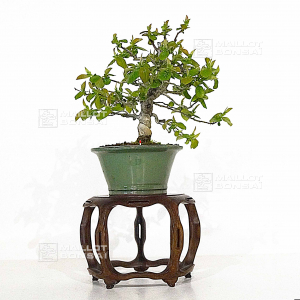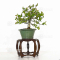##### The Japanese Bonsai specialist
Direct order Contact Help / Services Newsletter# Pseudo cydonia sinensis ref: 24030221

Pseudo cydonia sinensisref. : 11633

185,00

Available quantity : 1Order

###### Description

Height of the tree only: 220 mm. Width : 280 mm.

Dimensions of the enameled pot: 170*85 mm. Moyogi style. This bonsaï comes from seedlings and does not have strong wire or grafting marks or strong cutting wounds.  The Copper-colored foliage during spring turns green during summer and bright orange-toned yellow during autumn.

The bark desquamates and lets appear a superb trunk. Pinky white flowering during spring and yellow fruits at the end of summer.

The good conicity of the trunk, the ramifications, the beauty, the formed bark, and the soft movement of the trunk are characteristics that make this bonsaï a quality subject. Diameter of the trunk: about 20/25 mm.

Photographed in March 2022. This piece comes from master Takahashi's nursery.

Shelf not included.

Cut and repoted in September 2021.

#during 4.6 #bonsai 4.6 #trunk 4.5 #this 3.4 #sinensis 2.8 #outdoor 2.7 #cydonia 2.7 #yellow 2.6 #pseudo 2.6 #summer 2.6

Formule
(( ROUND((CHAR_LENGTH(b.article_nom)-CHAR_LENGTH(REPLACE(b.article_nom, 'during', '')))/LENGTH('during')) + ROUND((CHAR_LENGTH(b.article_description)-CHAR_LENGTH(REPLACE(b.article_description, 'during', '')))/LENGTH('during')) ) * 4.6) + (( ROUND((CHAR_LENGTH(b.article_nom)-CHAR_LENGTH(REPLACE(b.article_nom, 'trunk', '')))/LENGTH('trunk')) + ROUND((CHAR_LENGTH(b.article_description)-CHAR_LENGTH(REPLACE(b.article_description, 'trunk', '')))/LENGTH('trunk')) ) * 4.5) + (( ROUND((CHAR_LENGTH(b.article_nom)-CHAR_LENGTH(REPLACE(b.article_nom, 'this', '')))/LENGTH('this')) + ROUND((CHAR_LENGTH(b.article_description)-CHAR_LENGTH(REPLACE(b.article_description, 'this', '')))/LENGTH('this')) ) * 3.4) + (( ROUND((CHAR_LENGTH(b.article_nom)-CHAR_LENGTH(REPLACE(b.article_nom, 'sinensis', '')))/LENGTH('sinensis')) + ROUND((CHAR_LENGTH(b.article_description)-CHAR_LENGTH(REPLACE(b.article_description, 'sinensis', '')))/LENGTH('sinensis')) ) * 2.8) + (( ROUND((CHAR_LENGTH(b.article_nom)-CHAR_LENGTH(REPLACE(b.article_nom, 'cydonia', '')))/LENGTH('cydonia')) + ROUND((CHAR_LENGTH(b.article_description)-CHAR_LENGTH(REPLACE(b.article_description, 'cydonia', '')))/LENGTH('cydonia')) ) * 2.7) + (( ROUND((CHAR_LENGTH(b.article_nom)-CHAR_LENGTH(REPLACE(b.article_nom, 'yellow', '')))/LENGTH('yellow')) + ROUND((CHAR_LENGTH(b.article_description)-CHAR_LENGTH(REPLACE(b.article_description, 'yellow', '')))/LENGTH('yellow')) ) * 2.6) + (( ROUND((CHAR_LENGTH(b.article_nom)-CHAR_LENGTH(REPLACE(b.article_nom, 'pseudo', '')))/LENGTH('pseudo')) + ROUND((CHAR_LENGTH(b.article_description)-CHAR_LENGTH(REPLACE(b.article_description, 'pseudo', '')))/LENGTH('pseudo')) ) * 2.6) + (( ROUND((CHAR_LENGTH(b.article_nom)-CHAR_LENGTH(REPLACE(b.article_nom, 'summer', '')))/LENGTH('summer')) + ROUND((CHAR_LENGTH(b.article_description)-CHAR_LENGTH(REPLACE(b.article_description, 'summer', '')))/LENGTH('summer')) ) * 2.6) + (( ROUND((CHAR_LENGTH(b.article_nom)-CHAR_LENGTH(REPLACE(b.article_nom, 'strong', '')))/LENGTH('strong')) + ROUND((CHAR_LENGTH(b.article_description)-CHAR_LENGTH(REPLACE(b.article_description, 'strong', '')))/LENGTH('strong')) ) * 2.6) + (( ROUND((CHAR_LENGTH(b.article_nom)-CHAR_LENGTH(REPLACE(b.article_nom, 'spring', '')))/LENGTH('spring')) + ROUND((CHAR_LENGTH(b.article_description)-CHAR_LENGTH(REPLACE(b.article_description, 'spring', '')))/LENGTH('spring')) ) * 2.6)

## Secure payment## Delivery

Our logistic partners :04 74 55 23 48
Pépinière MAILLOT-BONSAÏ
Le Bois Frazy
01990 RELEVANT - FRANCE
on appointment